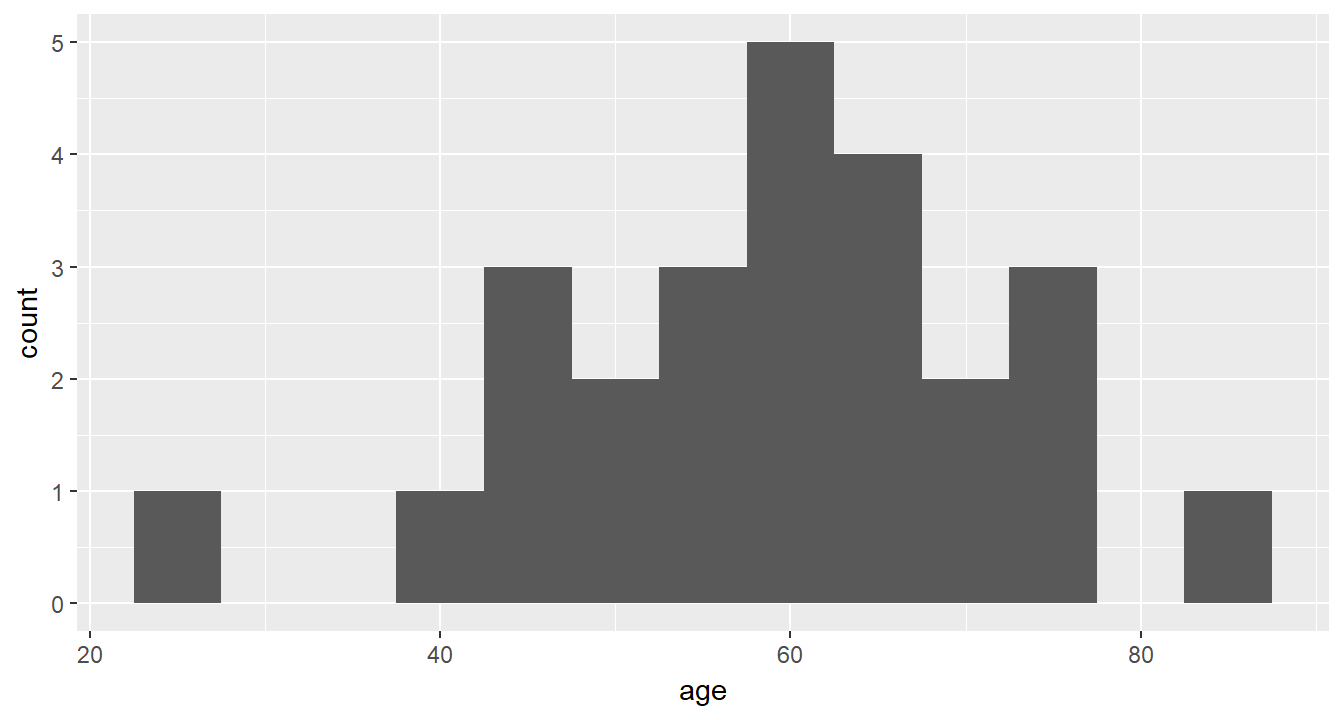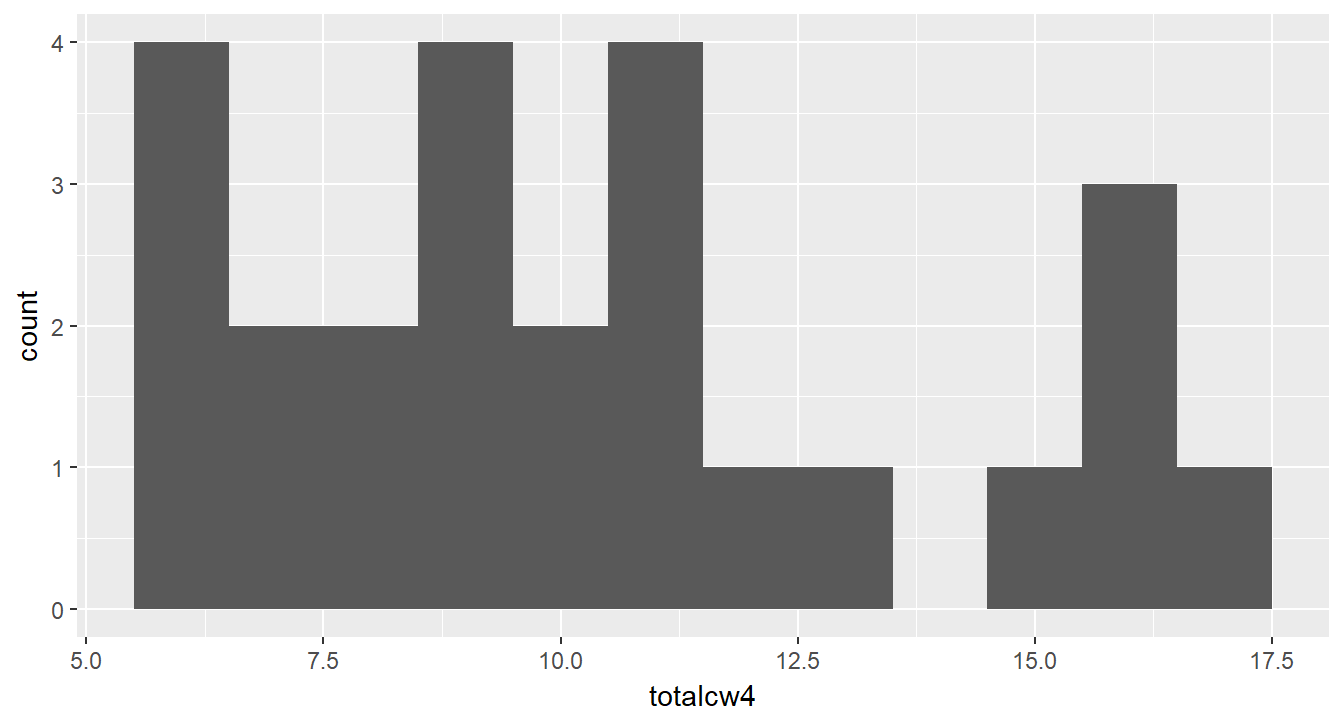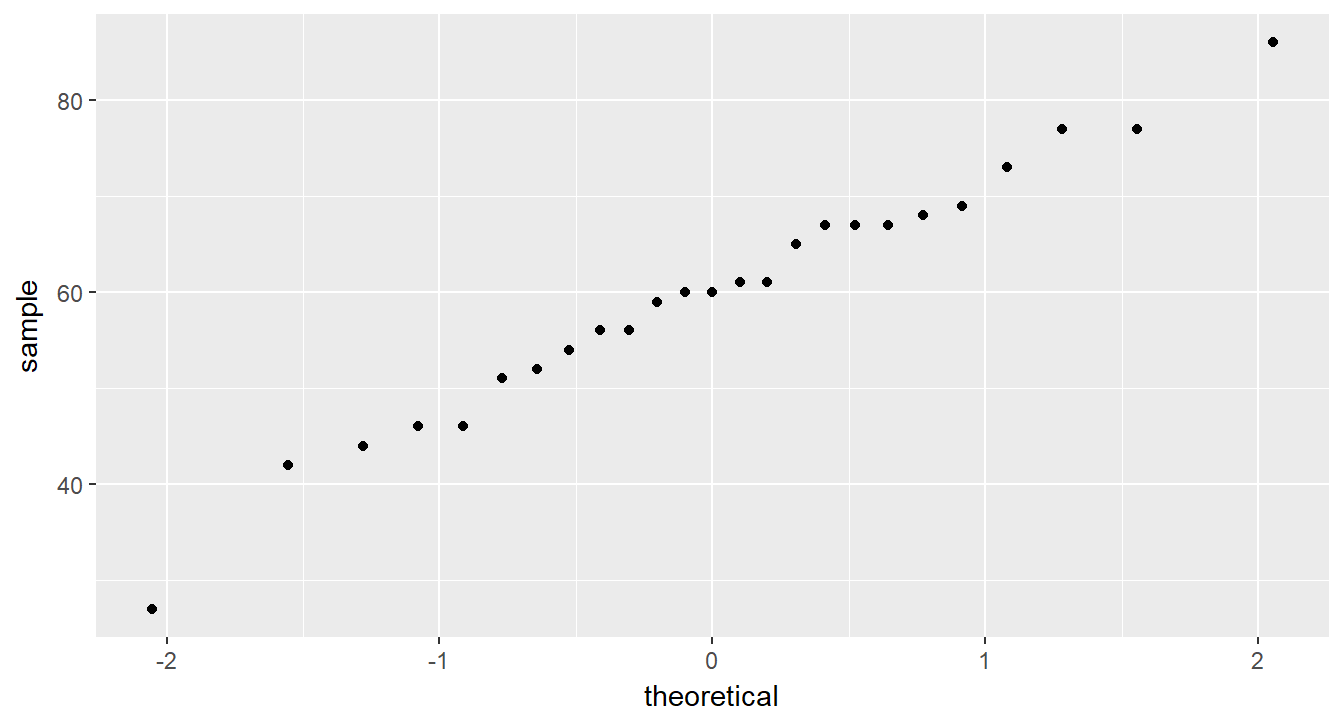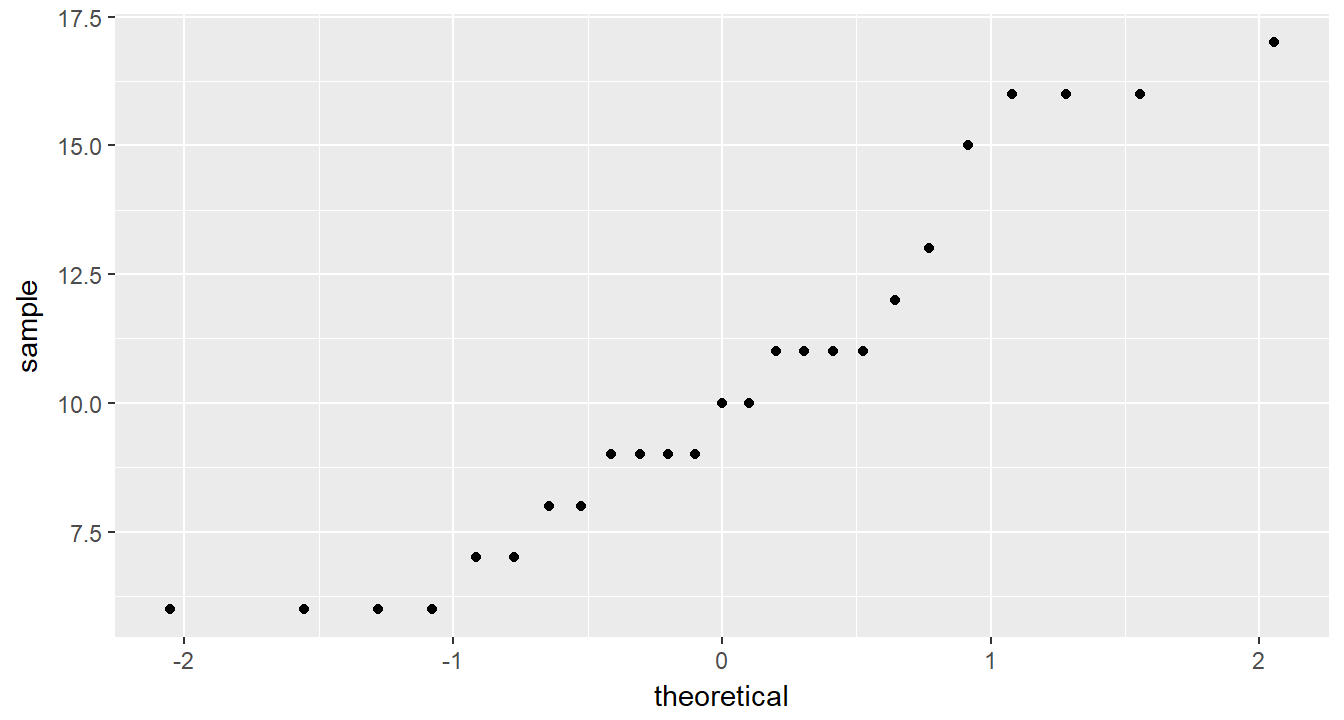# Chapter 5 TESTING NORMALITY

Required Packages

library(tidyverse)    # Loads several very helpful 'tidy' packages
library(haven)        # Read in SPSS datasets
library(psych)        # Lots of nice tid-bits

Example: Cancer Experiment

The Cancer dataset was introduced in chapter 3.

## 5.1 Skewness & Kurtosis

The psych::describe() function may be used to calculate skewness and kurtosis.

cancer_clean %>%
dplyr::select(age, totalcw4) %>%
psych::describe()
         vars  n  mean    sd median trimmed   mad min max range  skew
age         1 25 59.64 12.93     60   59.95 11.86  27  86    59 -0.31
totalcw4    2 25 10.36  3.47     10   10.19  2.97   6  17    11  0.49
kurtosis   se
age         -0.01 2.59
totalcw4    -1.00 0.69

## 5.2 Shapiro-Wilk’s Test

The shapiro.test() function is used to test for normality in a small’ish sample. This function is meant to be applied to a single variable in vector form, thus precede it with a dplyr::pull() step.

If $$p-value \gt \alpha$$, then the sample does NOT provide evidence the population is non-normally distributed.

cancer_clean %>%
dplyr::pull(age) %>%
shapiro.test()

Shapiro-Wilk normality test

data:  .
W = 0.98317, p-value = 0.9399

If $$p-value \lt \alpha$$, then the sample DOES provide evidence the population is non-normally distributed.

cancer_clean %>%
dplyr::pull(totalcw4) %>%
shapiro.test()

Shapiro-Wilk normality test

data:  .
W = 0.9131, p-value = 0.03575

## 5.3 Histogram

Histograms provide a visual way to determine if a data are approximately normally distributed. Look for a ‘bell’ shape.

cancer_clean %>%
ggplot(aes(age)) +
geom_histogram(binwidth = 5)cancer_clean %>%
ggplot(aes(totalcw4)) +
geom_histogram(binwidth = 1)## 5.4 Q-Q Plot

Quantile-quantile plots also help visually determine if data are approximately normally distributed. Look for the points to fall on a straight $$45 \degree$$ line.

cancer_clean %>%
ggplot(aes(sample = age)) +
geom_qq()cancer_clean %>%
ggplot(aes(sample = totalcw4)) +
geom_qq()